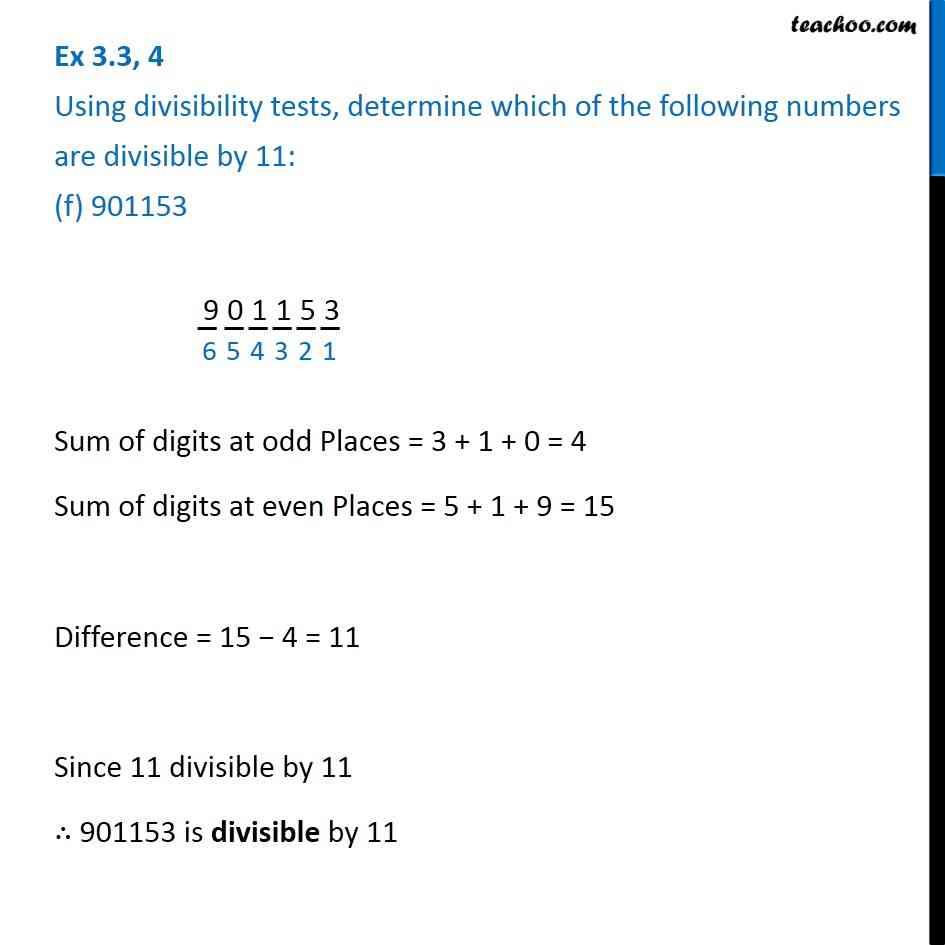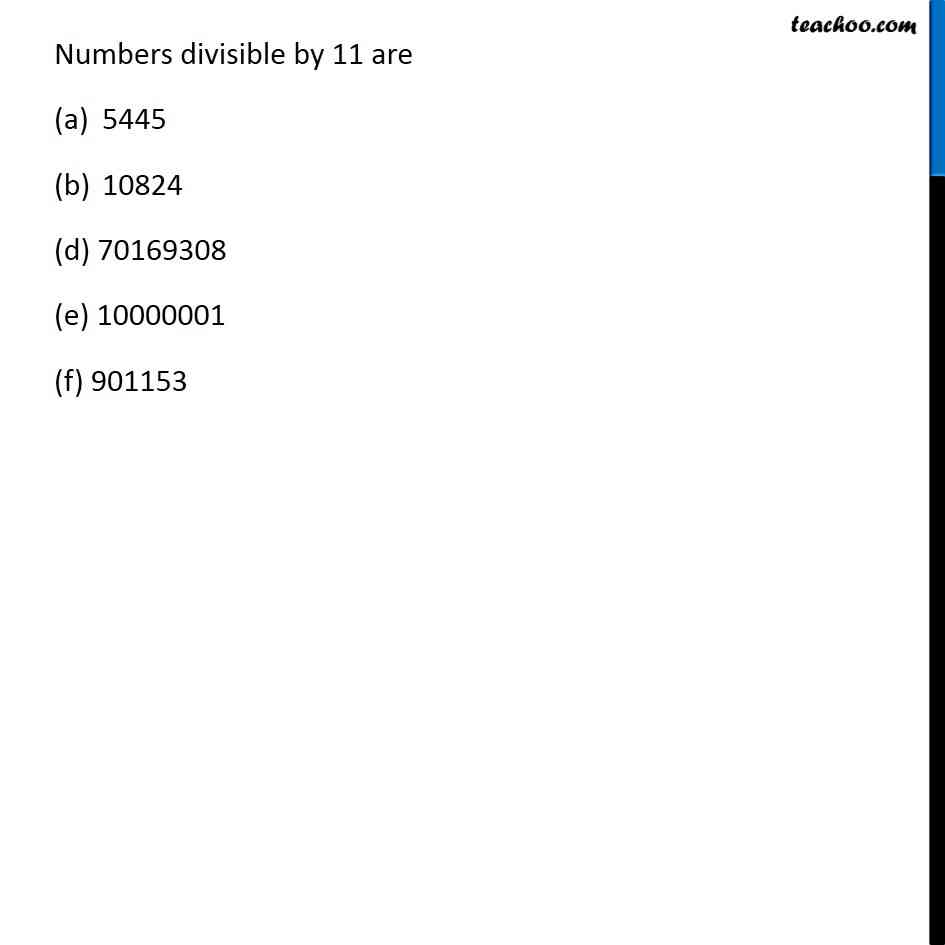Ex 3.3

Chapter 3 Class 6 Playing with Numbers
Serial order wiseLearn in your speed, with individual attention - Teachoo Maths 1-on-1 Class

### Transcript

Ex 3.3, 4 Using divisibility tests, determine which of the following numbers are divisible by 11: (f) 901153 901153 Sum of digits at odd Places = 3 + 1 + 0 = 4 Sum of digits at even Places = 5 + 1 + 9 = 15 Difference = 15 − 4 = 11 Since 11 divisible by 11 ∴ 901153 is divisible by 11 Numbers divisible by 11 are 5445 10824 (d) 70169308 (e) 10000001 (f) 901153